### Preliminary Observations

Figure-1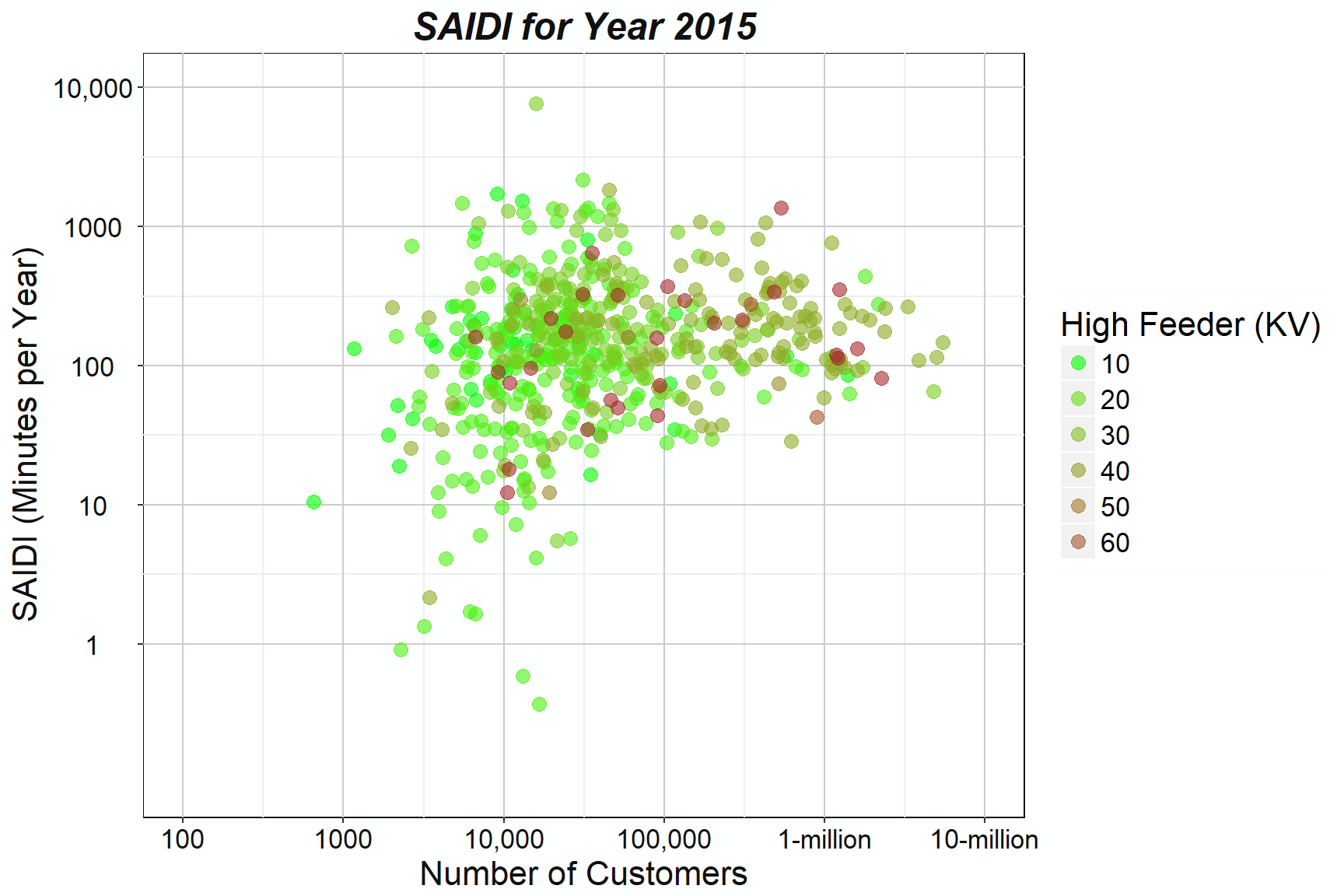## Exploring the Power Outage Events

Figure-2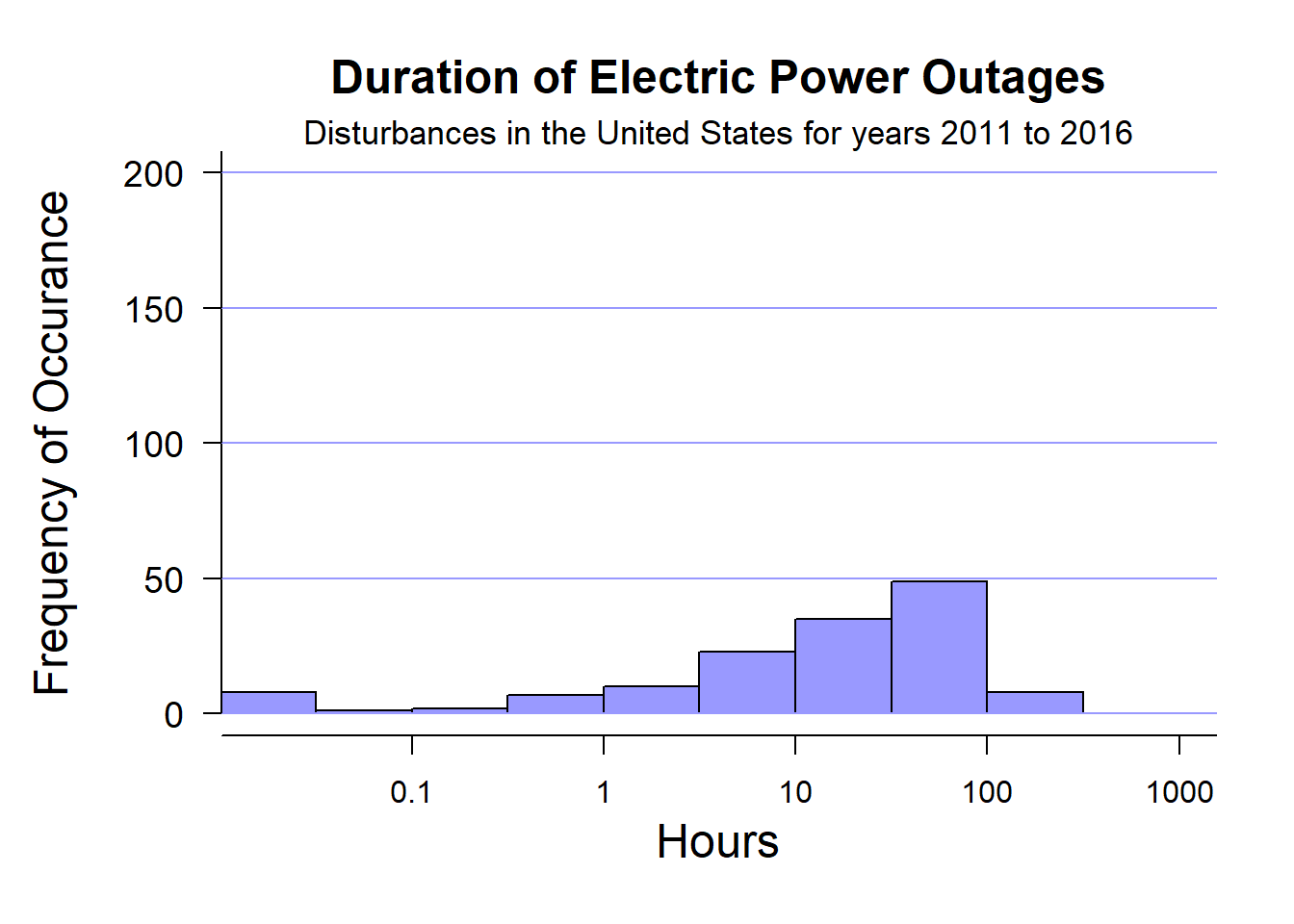paste(formatC(sum(timedat$Duration)/(dim(timedat))), digits=3, "-hours", sep="") ##  "34.653-hours" ##### The vast majority of outages last no more than one week (168-hours). Figure-3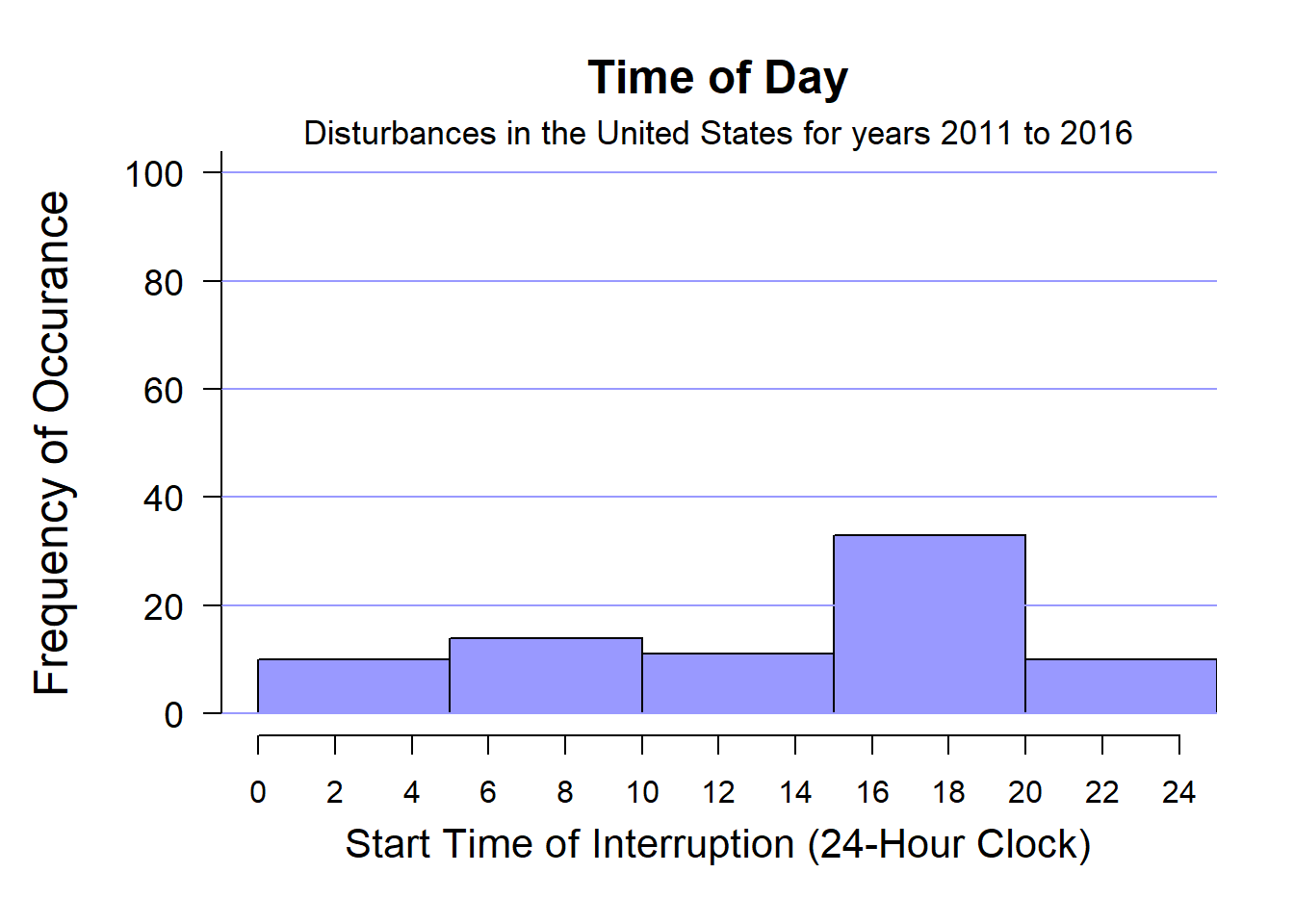##### The plot above shows that the probability of a disruption roughly doubles between 4:00 PM (16-hours) and 8:00 PM (20-hours). The problems are the least in the late morning after 10:00 AM. This data indicates that most problems are random systematic ones or weather related. ### Calculating SAIDI ##### Let’s assume that there were three disturbances during the year at a utility with durations of 10 hours, 20 hours, and 60 hours that in turn affected 30,000, 20,000, and 8,000 customers out of a total of 1 million customers. ##### $SAIDI = \frac {10 \cdot 30000 + 20 \cdot 20000 + 60 \cdot 8000}{1,000,000}$ ##### $$U_1 = 10$$ hours; $$U_2 = 20$$ hours; $$U_3 = 60$$ hours ##### $$N_1 = 30,000$$ customers; $$N_2 = 20,000$$ customers; $$N_i = 8,000$$ customers ##### $$N_y = 3$$ events; $$N_T = 1,000,000$$ total customers SAIDI <- ((10 * 30000) + (20 * 20000) + (60 * 8000))/ 1000000 paste ("SAIDI = ", SAIDI, "-hours",sep="") ##  "SAIDI = 1.18-hours" ### A different example using minutes instead of hours: ##### $$U_1 = 98$$ minutes, $$U_2 = 21$$ minutes, $$U_3 = 95$$ minutes ##### $$N_1 = 2750$$ impacted customers, $$N_2 = 1710$$ impacted customers, $$N_i = 1516$$ impacted customers ##### $$N_y = 3$$ events ##### $$N_T = 84500$$ total customers SAIDI <- ((98 * 2750) + (21 * 1710) + (95 * 1516))/ 84500 paste ("SAIDI = ", formatC(SAIDI, digits=3), "-minutes",sep="") ##  "SAIDI = 5.32-minutes" ### SAIFI: Frequency of Interruptions ##### SAIFI is an acronym for System Average Interruption Frequency Index (SAIFI) and indicates the average number of times that customers experience outages, usually over one-year. The SAIFI figure could depend on how it is measured unless you could keep track of how many times every customer loses power. Let $$\lambda_i$$ be the average frequency of interruptions over a one year period in areas that we call “neighborhoods” which are small enough so that most people within one lose power together when disruptions occur. We call $$\lambda_i$$ the failure rate. Let $$N_i$$ be the number of customers in each neighborhood and $$N$$ be the number of neighborhoods for the entire area served by the utility. ##### The failure rate and the number of customers in each neighborhood are multiplied together and summed over all neighborhoods. Finally, the sum is divided by the total number of customers $$N_T$$. #### $SAIFI = \frac {\sum_{i=1}^{N} {\lambda_i \cdot N_i}}{N_T}$ ### Calculating SAIFI ##### Let’s assume that there were three neighborhoods which respectively experienced 5, 4, and 2 interruptions (failures) during the year in regions of 1000, 2000. and 7000 customers. This corresponds to failure rates of 5, 4, and 2 for the year. #### $SAIFI = \frac {5 \cdot 1000 + 4 \cdot 2000 + 2 \cdot 7000}{10,000}$ ##### $$\lambda_1 = 5$$ failures; $$\lambda_2 = 4$$ failures; $$\lambda_3 = 2$$ failures ##### $$N_1 = 1000$$ impacted customers; $$N_2 = 2000$$ impacted customers; $$N_3 = 7000$$ impacted customers ##### $$N_y = 3$$ regions; N_T = 10,000$ total customers
SAIFI <- ((5 * 1000) + (4 * 2000) + (2 * 7000))/ 10000
paste ("SAIFI = ", SAIFI, " Events per year",sep="")
##  "SAIFI = 2.7 Events per year"

### Weather - The most Significant Problem

##### The significant factor for power disruptions is the weather. The other reasons are small by comparison by more than an order of magnitude.
Figure-4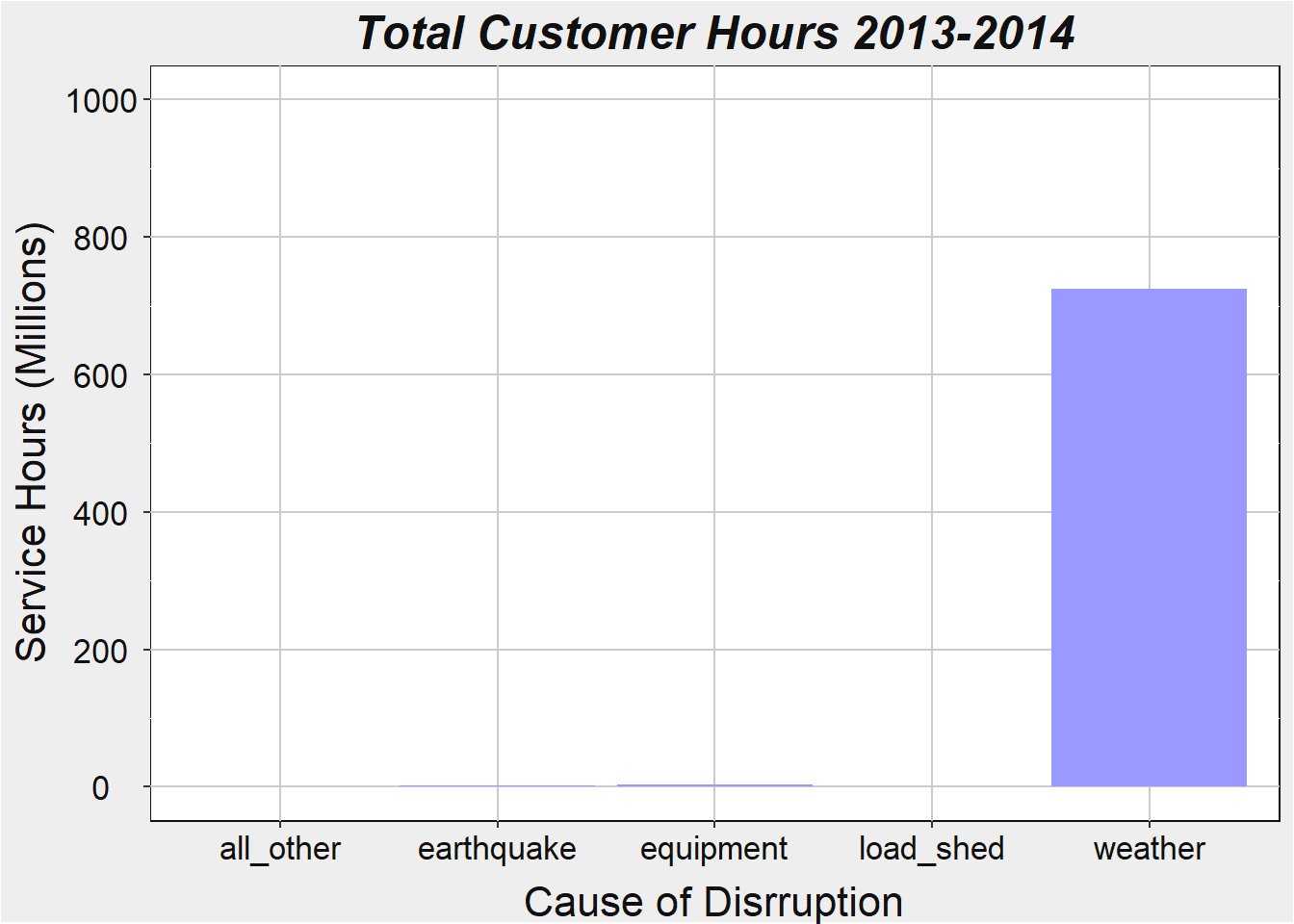Figure-5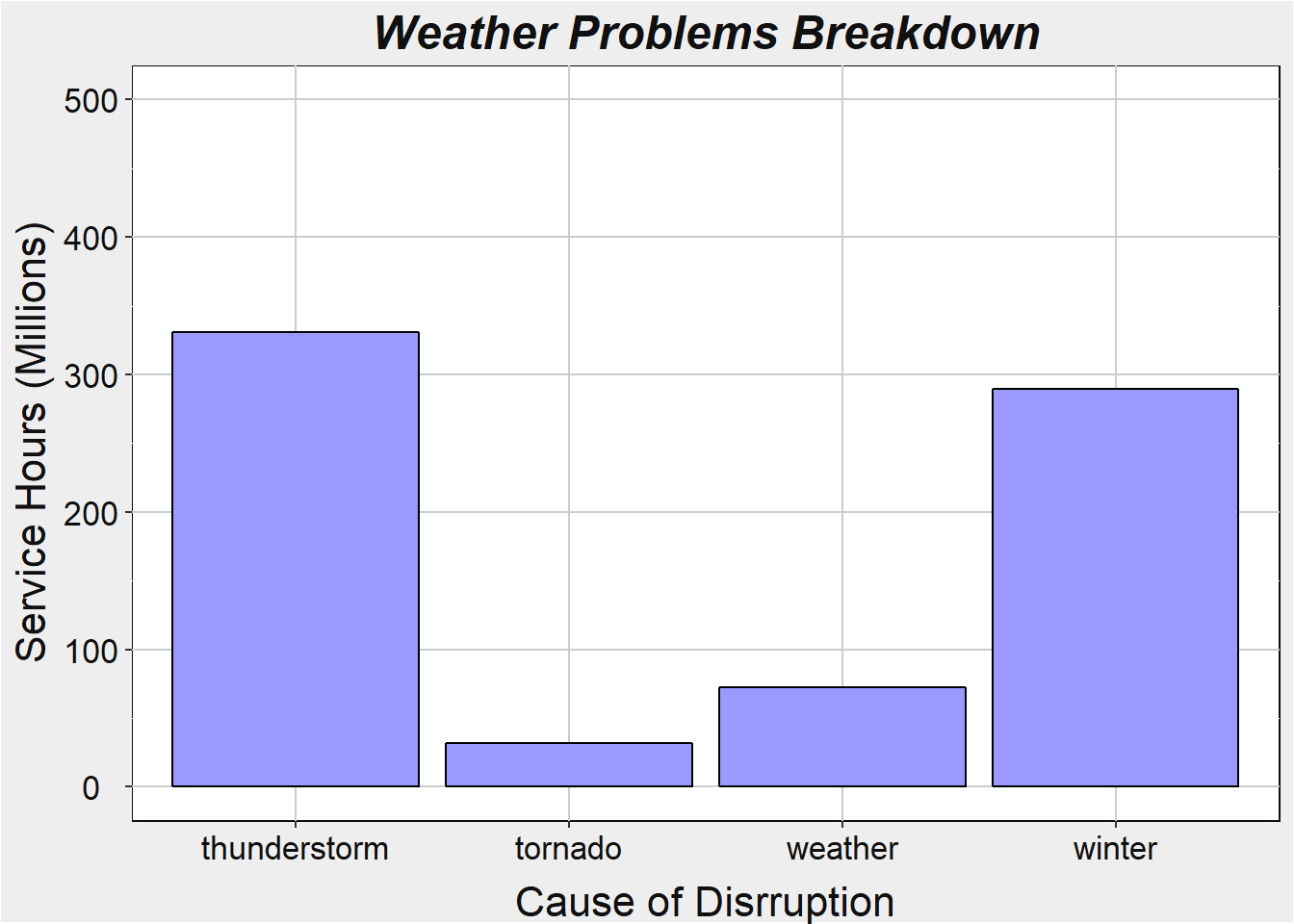Figure-6